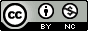## Wednesday, May 13, 2009

### Don't categorize continuous variables!

If you're doing an analysis with variables that naturally vary on a continuous scale, like age or smoking pack-years, NEVER be tempted to categorize individuals into groups - there's nearly always a better approach that utilizes the full distribution of values. It may seem convenient for a particular analysis you're doing but you'll take an enormous hit in power and precision. Frank Harrell in Vanderbilt's Biostatistics department wrote an excellent list of reasons why this is a terrible idea.

For an interactive example, take 5 seconds to look at this applet that illustrates the problem. Look at the t-statistic for the regression coefficient, and especially the R-squared. Moving the slider to the right simulates splitting observations at the median of X into two categories. The regression coefficient fluctuates, but both the t-statistic and the R-squared shrink substantially - enough to make the result no longer significant. Here's what it looks like:

Using the full distribution of your data:
b=.43 ; p=.036 ; R²=.221After a median split:
b=.29 ; p=.275 ; R²=.066The bottom line here is that if you have continuous variables, pick an analysis method that doesn't discard useful variation in your data! See the two previous posts (part I and part II) for help choosing the best method for the data types you have.

#### 1 comment:

1.Nice, thank you